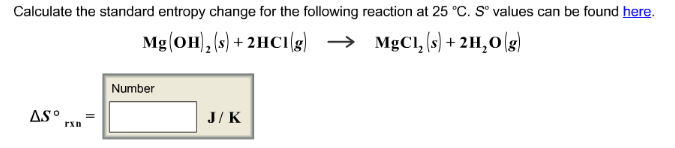# Problem: Calculate the standard entropy change for the following reaction at 25°C. S° values can be found here. Mg(OH)2 (s) + 2HCl (g) → MgCl2 (s) + 2H2O (g)

###### FREE Expert Solution
96% (29 ratings)###### Problem Details

Calculate the standard entropy change for the following reaction at 25°C. S° values can be found here.

Mg(OH)2 (s) + 2HCl (g) → MgCl2 (s) + 2H2O (g)